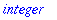Error: invalid arguments

Many of Maple's commands and functions are very particular about the number, types and order of the arguments they are given, and will report an error if these are not correct.

Look at the help for a function, in particular the "calling sequence" and "parameters" sections, to see the correct forms to use. Or imitate one of the examples that are given on the help page.

Use the
tracelast command to see more about the error. In particular it will show what functions were being called with what arguments when the error occurred. In some cases it may be helpful to use the type or whattype command to check on the type of some argument.

One common way that these errors can occur is when you try to use a name as a symbolic variable, but it turns out that the name has been assigned a value. This is the case in the second example below. If so, either use a different name that does not have a value or unassign the value if it is no longer needed.

Examples:

> limit([x,x^2],x=1);

Error, (in limit) invalid arguments

> p:= 5:

> fsolve(p^2=2,p);

Error, (in fsolve) invalid arguments

> tracelast;

Error, (in fsolve) invalid arguments

executing statement: ERROR(`invalid arguments`)

locals defined as: _X = _X, eqns = eqns, opt = opt, r = r, sol = sol, tvars = tvars, s = s, t = t

fsolve called with arguments: 25 = 1, 5

> whattype(p);